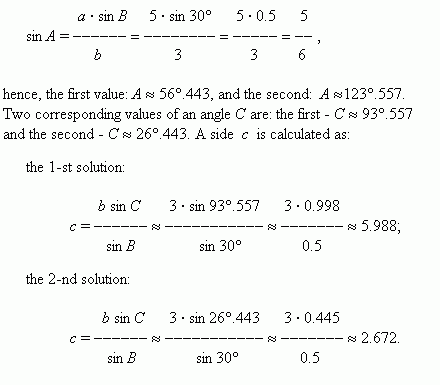# Solving of oblique triangles

 Case 1. Three sides a, b, c are given. Find angles A, B, C. By the law of cosines we find one of the angles: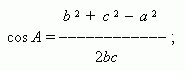the second angle we find by the law of sines: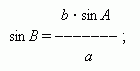the third angle is found by the formula: C = 180° – ( A + B ).

 E x a m p l e . Three sides of a triangle are given: a = 2, b = 3, c = 4. Find angles of this triangle. S o l u t i o n .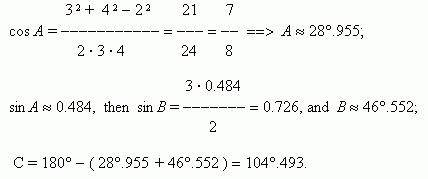Case 2. Given: two sides a and b and angle C between them. Find a side c and angles A and B . By the law of cosines we find a side c : c 2 = a 2 +  b 2 - 2 ab · cos C ; and then by the law of sines – an angle A :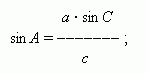here it is necessary to emphasize that A is an acute angle, if b / a > cos C , and an obtuse angle, if b / a < cos C . The third angle B = 180° – ( A + C ).

 Case 3. Any two angles and a side are given. Find the third angle and two other sides. It is obvious, that the third angle is calculated by the formula: A+ B+ C = 180°, and then using the law of sines we find two other sides.

 Case 4. Given two sides a and b and angle B , opposite one of them. Find a side c and angles A and C . At first by the law of sines we find an angle A :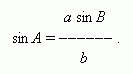The following cases are possible here: 1) a > b ; a · sin B > b –  there is no solution here; 2) a > b ; a · sin B = b –  there is one solution here, A is a right angle; 3) a > b ; a · sin B < b < a  – there are two solutions here: A may be taken either an acute or an obtuse angle; 4) a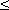b –  there is one solution here, A – an acute angle. After determining an angle A , we find the third angle: C = 180° – ( A+ B ). Ii is obvious that if A can have two values, then also C can have two values. Now the third side can be determined by the law of sines: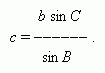If  we found two values for C , then also a side c has two values, hence, two different triangles satisfy  the given conditions.

 E x a m p l e . Given: a = 5, b = 3, B = 30°. Find a side c and angles A and C . S o l u t i o n . We have here: a > b and a sin B < b . ( Check it please ! ). Hence, according to the case 3  two solutions are possible here: